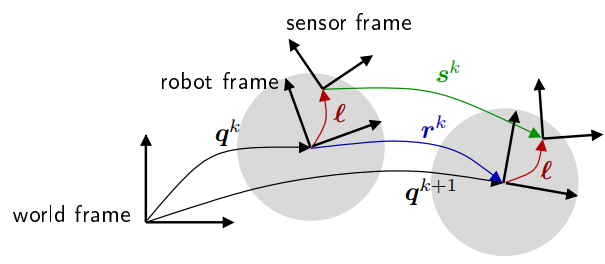# 2d Laser 和 Odomter 内外参数标定工具原理及使用方法

## 标定原理

### 原理概述( v ω ) = J ( ω L ω R ) \left( \begin{array}{l}{v} \\ {\omega}\end{array}\right)=\boldsymbol{J} \left( \begin{array}{c}{\omega_{\mathrm{L}}} \\ {\omega_{\mathrm{R}}}\end{array}\right)

J = ( J 11 J 12 J 21 J 22 ) = ( + r L / 2 + r R / 2 − r L / b + r R / b ) \boldsymbol{J}=\left( \begin{array}{ll}{J_{11}} &amp; {J_{12}} \\ {J_{21}} &amp; {J_{22}}\end{array}\right)=\left( \begin{array}{cc}{+r_{\mathrm{L}} / 2} &amp; {+r_{\mathrm{R}} / 2} \\ {-r_{\mathrm{L}} / b} &amp; {+r_{\mathrm{R}} / b}\end{array}\right)

s k = ⊖ ( q k ⊕ ℓ ) ⊕ ( q k + 1 ⊕ ℓ )         ( 1 ) \boldsymbol{s}^{k}=\ominus\left(\boldsymbol{q}^{k} \oplus \boldsymbol{\ell}\right) \oplus\left(\boldsymbol{q}^{k+1} \oplus \boldsymbol{\ell}\right) ~~~~~~~ (1)

( a x a y a θ ) ⊕ ( b x b y b θ ) = ( a x + b x cos ⁡ ( a θ ) − b y sin ⁡ ( a θ ) a y + b x sin ⁡ ( a θ ) + b y cos ⁡ ( a θ ) a θ + b θ ) \left( \begin{array}{l}{a_{x}} \\ {a_{y}} \\ {a_{\theta}}\end{array}\right) \oplus \left( \begin{array}{l}{b_{x}} \\ {b_{y}} \\ {b_{\theta}}\end{array}\right)=\left( \begin{array}{c}{a_{x}+b_{x} \cos \left(a_{\theta}\right)-b_{y} \sin \left(a_{\theta}\right)} \\ {a_{y}+b_{x} \sin \left(a_{\theta}\right)+b_{y} \cos \left(a_{\theta}\right)} \\ {a_{\theta}+b_{\theta}}\end{array}\right)
⊖ ​ \ominus​ 表示求逆：
⊖ ( a x a y a θ ) = ( − a x cos ⁡ ( a θ ) − a y sin ⁡ ( a θ ) + a x sin ⁡ ( a θ ) − a y cos ⁡ ( a θ ) − a θ ) \ominus \left( \begin{array}{l}{a_{x}} \\ {a_{y}} \\ {a_{\theta}}\end{array}\right)=\left( \begin{array}{c}{-a_{x} \cos \left(a_{\theta}\right)-a_{y} \sin \left(a_{\theta}\right)} \\ {+a_{x} \sin \left(a_{\theta}\right)-a_{y} \cos \left(a_{\theta}\right)} \\ {-a_{\theta}}\end{array}\right)

s k = ⊖ ℓ ⊕ r k ( r L , r R , b ) ⊕ ℓ         ( 2 ) \boldsymbol{s}^{k}=\ominus \boldsymbol{\ell} \oplus \boldsymbol{r}^{k}\left(r_{\mathrm{L}}, r_{\mathrm{R}}, b\right) \oplus \boldsymbol{\ell}~~~~~~~(2)

J = − 1 2 ∑ k = 1 n ∥ s ^ k − ⊖ ℓ ⊕ r k ( r L , r R , b ) ⊕ ℓ ∥ Σ k − 1 2         ( 3 ) \mathcal{J}=-\frac{1}{2} \sum_{k=1}^{n}\left\|\hat{\boldsymbol{s}}^{k}-\ominus \ell \oplus \boldsymbol{r}^{k}\left(r_{\mathrm{L}}, r_{\mathrm{R}}, b\right) \oplus \ell\right\|_{\mathbf{\Sigma}_{k}^{-1}}^{2} ~~~~~~~(3)

### 标定算法的具体实现

1. 先求线性部分：首先由于激光坐标系和机器人坐标系都是水平面运动，所以两个传感器得到的两个时刻之间的角度变化是相等的 s θ k = r θ k \boldsymbol{s}_{\theta}^{k}=\boldsymbol{r}_{\theta}^{k} . 因此直接利用公式（2）中的对应项构建等式，用线性最小二乘直接求出轮速计的内参数矩阵的两个元素：
( J ^ 21 J ^ 22 ) = [ ∑ k L k T L k ( σ θ k ) 2 ] − 1 ∑ k L k T ( σ θ k ) 2 s ^ θ k \left( \begin{array}{c}{\hat{J}_{21}} \\ {\hat{J}_{22}}\end{array}\right)=\left[\sum_{k} \frac{\boldsymbol{L}_{k}^{T} \boldsymbol{L}_{k}}{\left(\sigma_{\theta}^{k}\right)^{2}}\right]^{-1} \sum_{k} \frac{\boldsymbol{L}_{k}^{T}}{\left(\sigma_{\theta}^{k}\right)^{2}} \hat{s}_{\theta}^{k}
具体公式可以参照论文。

2. 再闭式求解非线性部分：有了部分线性内参数，作者对公式（3）进行了简单的变形，变成了 J = − 1 2 ∑ k ∥ ℓ ⊕ s ^ k − r k ⊕ ℓ ∥ Σ k − 1 2 \mathcal{J}=-\frac{1}{2} \sum_{k}\left\|\boldsymbol{\ell} \oplus \hat{\boldsymbol{s}}^{k}-\boldsymbol{r}^{k} \oplus \boldsymbol{\ell}\right\|_{\mathbf{\Sigma}_{k}^{-1}}^{2} . 这样这个公式就能转化成带约束的二次型问题，然后利用拉格朗日乘数转化成闭式解。具体的推导请参看论文。

3. outlier remove : 采样的数据中可能有轮子打滑等无效数据在里面，会降低标定精度，因此标定过程反复迭代多次，每迭代一次，就计算一下每个采样数据的 Chi-2 误差。
χ k = ∥ s ^ k − ⊖ ℓ ^ ⊕ r k ( r ^ L , r ^ R , b ^ ) ⊕ ℓ ^ ∥ Σ k − 1 \chi^{k}=\left\|\hat{\boldsymbol{s}}^{k}-\ominus \hat{\ell} \oplus \boldsymbol{r}^{k}\left(\hat{r}_{\mathrm{L}}, \hat{r}_{\mathrm{R}}, \hat{b}\right) \oplus \hat{\ell}\right\|_{\mathbf{\Sigma}_{k}^{-1}}
然后根据误差大小排序，丢弃掉一定百分比的数据。

4. 协方差估计：协方差的估计直接采用信息矩阵的逆来计算。如果给定一个模型 y k = f k ( x ) + ϵ k \boldsymbol{y}_{k}=\boldsymbol{f}_{k}(\boldsymbol{x})+\boldsymbol{\epsilon}_{k} 其中 f \boldsymbol{f} 是可导函数， ϵ k \boldsymbol{\epsilon}_{k} 是协方差为 Σ k \mathbf{\Sigma}_{k} 的高斯噪声。协方差的下界 Cramer-Rao bound， cov ⁡ ( x ^ ) ≥ I ( x ) − 1 \operatorname{cov}(\hat{\boldsymbol{x}}) \geq \mathcal{I}(\boldsymbol{x})^{-1} . 在这个应用中， x = ( r R , r L , b , ℓ x , ℓ y , ℓ θ ) \boldsymbol{x}=\left(r_{\mathrm{R}}, r_{\mathrm{L}}, b, \ell_{x}, \ell_{y}, \ell_{\theta}\right) y k = s ^ k \boldsymbol{y}_{k}=\hat{\boldsymbol{s}}^{k} ，观测函数 f k \boldsymbol{f}_{k} 为公式（2）所示。这个模型最小二乘估计的参数 x \boldsymbol{x} 的信息矩阵为

I ( x ) = ∑ k ∂ f k ∂ x Σ k − 1 ∂ f k ∂ x \mathcal{I}(\boldsymbol{x})=\sum_{k} \frac{\partial \boldsymbol{f}_{k}}{\partial \boldsymbol{x}} \boldsymbol{\Sigma}_{k}^{-\mathbf{1}} \frac{\partial \boldsymbol{f}_{k}}{\partial \boldsymbol{x}}

## 工具使用

   cd catkin_ws/src
git clone https://github.com/MegviiRobot/OdomLaserCalibraTool
cd ..
catkin_make
source devel/setup.bash
roslaunch example.launch



12-261000
12-22
02-26169
07-181178
07-295952
04-201万+
06-274424
02-181935
10-226138
10-283151
09-183058
09-146968

### “相关推荐”对你有帮助么？

•非常没帮助
•没帮助
•一般
•有帮助
•非常有帮助被折叠的  条评论 为什么被折叠?到【灌水乐园】发言¥2 ¥4 ¥6 ¥10 ¥20余额支付 (余额：-- )扫码支付获取中扫码支付点击重新获取扫码支付1.余额是钱包充值的虚拟货币，按照1:1的比例进行支付金额的抵扣。
2.余额无法直接购买下载，可以购买VIP、C币套餐、付费专栏及课程。余额充值